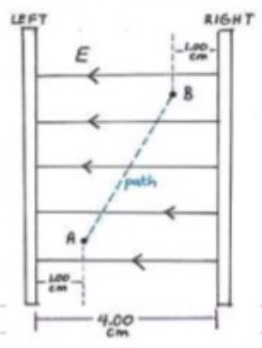# Problem: 1. Calculate the magnitude of the constant electric field that exists in the space between two parallel conducting plates. The plates are separated by 1.00 cm and there is a potential difference between them of 1.50x104 V2. The magnitude of the electric field produced by two parallel plates is 7.50x104 N/C. The plates are 4.00 cm apart from each other as illustrated.(a) Calculate the potential difference between the plates:(b) Point A is 1.00 cm from the left plate. Point B is 1.00 cm from the right plate. What is the potential difference between points A and B?(c) Which point, A or B, is at higher potential?(d) If the potential at A is 28.0 V, what is the potential at B?(e) A positive charge qo = +0.250 C is moved from A to B, following the illustrated diagonal path. Calculate the work done by the field during the displacement of this particle:(f) The work calculates in part (e) is either positive or negative. Explain why the sign of your calculation makes sense in this case:

###### FREE Expert Solution

This problem involves the relationship between electric field and potential difference.

Electric field:

$\overline{){{\mathbf{E}}}_{{\mathbf{x}}}{\mathbf{=}}{\mathbf{-}}\frac{\mathbf{∆}\mathbf{V}}{\mathbf{∆}\mathbf{x}}}$

83% (319 ratings)###### Problem Details

1. Calculate the magnitude of the constant electric field that exists in the space between two parallel conducting plates. The plates are separated by 1.00 cm and there is a potential difference between them of 1.50x104 V

2. The magnitude of the electric field produced by two parallel plates is 7.50x104 N/C. The plates are 4.00 cm apart from each other as illustrated.(a) Calculate the potential difference between the plates:

(b) Point A is 1.00 cm from the left plate. Point B is 1.00 cm from the right plate. What is the potential difference between points A and B?

(c) Which point, A or B, is at higher potential?

(d) If the potential at A is 28.0 V, what is the potential at B?

(e) A positive charge qo = +0.250 C is moved from A to B, following the illustrated diagonal path. Calculate the work done by the field during the displacement of this particle:

(f) The work calculates in part (e) is either positive or negative. Explain why the sign of your calculation makes sense in this case: